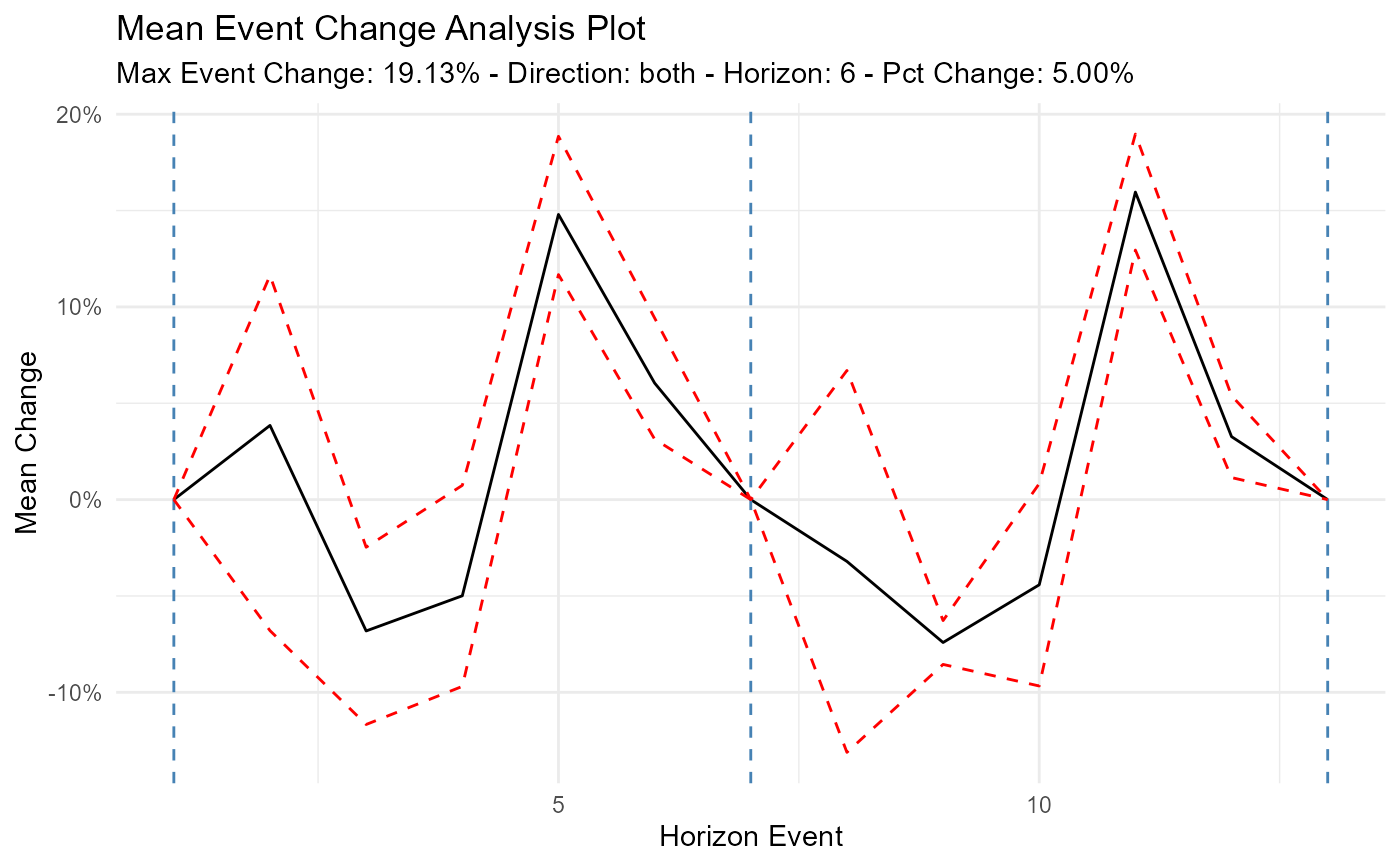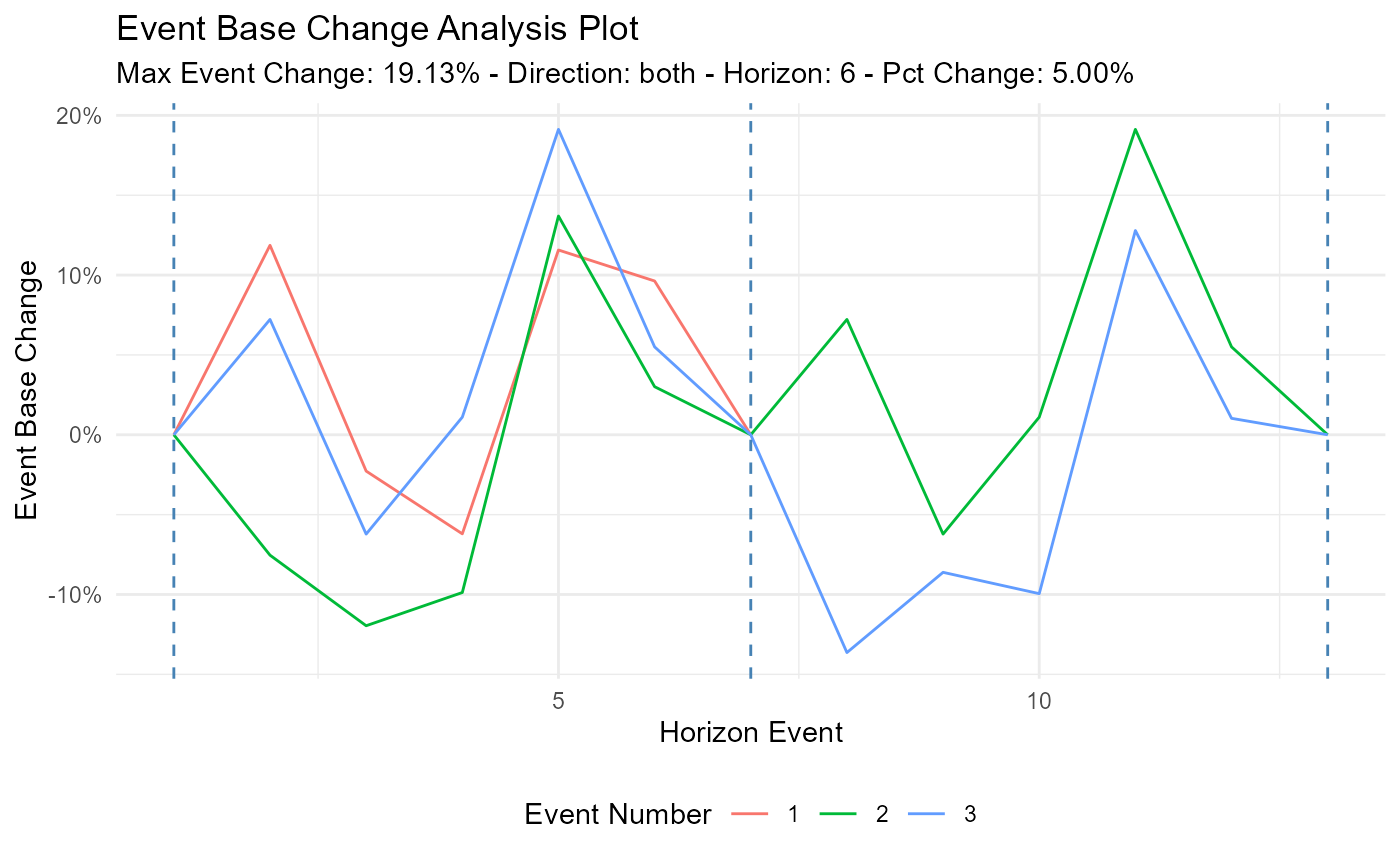Plot out the data from the ts_time_event_analysis_tbl() function.

## Usage

ts_event_analysis_plot(
.data,
.plot_type = "mean",
.plot_ci = TRUE,
.interactive = FALSE
)

## Arguments

.data

The data that comes from the ts_time_event_analysis_tbl()

.plot_type

The default is "mean" which will show the mean event change of the output from the analysis tibble. The possible values for this are: mean, median, and individual.

.plot_ci

The default is TRUE. This will only work if you choose one of the aggregate plots of either "mean" or "median"

.interactive

The default is FALSE. TRUE will return a plotly plot.

A ggplot2 object

## Details

This function will take in data strictly from the ts_time_event_analysis_tbl() and plot out the data. You can choose what type of plot you want in the parameter of .plot_type. This will give you a choice of "mean", "median", and "individual".

You can also plot the upper and lower confidence intervals if you choose one of the aggregate plots ("mean"/"median").

Other Plot: ts_brownian_motion_plot(), ts_qq_plot(), ts_scedacity_scatter_plot()

## Author

Steven P. Sanderson II, MPH

## Examples

library(dplyr)
df <- ts_to_tbl(AirPassengers) %>% select(-index)

ts_time_event_analysis_tbl(
.data = df,
.horizon = 6,
.date_col = date_col,
.value_col = value,
.direction = "both"
) %>%
ts_event_analysis_plot()ts_time_event_analysis_tbl(
.data = df,
.horizon = 6,
.date_col = date_col,
.value_col = value,
.direction = "both"
) %>%
ts_event_analysis_plot(.plot_type = "individual")